Published: 28 June 2021

# Improved 3D biomechanical model for evaluation of mass and inertial parameters in few body positions from NASA classification

Gergana Nikolova1
Mihail Tsveov2
Daniel Dantchev3
1, 2, 3, 4Institute of Mechanics, Bulgarian Academy of Sciences, Acad. G. Bonchev St., Bl. 4, Sofia 1113, Bulgaria
4University of National and World Economy, Student Town, UNWE, 1700 Sofia, Bulgaria
Corresponding Author:
Gergana Nikolova
Editor's Pick
Views 240

#### Abstract

The aim of the current article is: 1) to present a 20-segmental biomechanical model of the male human body generated within a SolidWorks® environment.; 2) to improve the 16-segmental biomechanical model of the human body described in our previous investigation, shaping the body with 20 instead of 16 segments.; 3) to determine the mass-inertial characteristics of the human body of the average Bulgarian male based on the model.; 3) to verify the proposed 3D CAD model of the human body against the analytical results from our previous investigation, as well as through comparison with data available in the provided reports.; 4) to predict a human body's mass and inertial properties in several body positions as classified by NASA. The comparison performed between our model results and data reported in the literature gives us confidence that this model could be reliably used to calculate these parameters at various postures of the body.

## 1. Introduction

In order to study human movement, we need to know the geometric and mass-inertial characteristics (MIC) such as volume, mass, the centre of mass, moments of inertia of human body (HB) segments in various body positions. Moments of inertia and centres of gravity of the body are among its fundamental characteristics. Definitely, these characteristics are required to perform computations, simulations, and predictions in various scientific areas such as biomechanics, biomedical engineering, medicine, ergonomics and sport. For example, in the field of anthropomorphic and rehabilitation robotics they are essential in the design of rehabilitation devices that aid the patient to perform a given movement; there the orthopaedic device should have appropriate geometry and suitable mass distribution. Obviously, the same is true in medicine and more particular in orthopaedics, traumatology, orthotics and prosthesis design. One of the modern directions of research where such knowledge is mandatory is the analysis of body motion under microgravity. There, the body rotation of an individual is easily produced by its own action or by external forces. In criminalistics, such information is necessary in order to study body fall or body impact (travel accidents) cases. Let us also mention the problem for the design of air or space transport systems, where human weight is a significant percentage of vehicle weight -, etc.

The field of human motion research, due to its importance, has been the subject of intensive simulations and mathematical modelling , . These sources also consider results on problems like the movement of segments of the HB during walking, running, the role of the muscles and skeleton system, etc.

The anthropometric lengths and data for moments of inertia and centres of gravity of male individuals of the Air Force crews reported by NASA  generated our interest in the problem. We wanted to find out to which extent NASA`s experimental data can be represented via mathematical modelling and how much data for an “average” astronaut contrast those for an “average” Bulgarian male. The methods presented in - and the results obtained there give a solid ground for the determination of the geometric and mass-inertial parameters of the HB and therefore we will closely follow the corresponding line of action.

The purpose of this study is to improve the 16-segmental biomechanical model of the human body described in our previous investigation , shaping the body with 20 instead of 16 segments. The model will be generated in a computer environment that allows not only the mass-inertial parameters of the single body segments to be calculated, which we have done also analytically, but to obtain these characteristics for the whole body in any position of interest. We devise a model of the human thigh that solves the long-standing problem in the mathematical modelling of the body achieving continuous tailoring of the thigh with the torso in a way that keeps, at the same time, the corresponding anthropometric angles as given in the experimental literature.

In this study, we present, in Section 2, a 20-segmental 3D model of the HB of the Bulgarian man. It allows for predicting the inertial properties of the HB in any fixed body position, including those provided in NASA’s classification. We obtain MIC, in Section 3, of an average Bulgarian male using SolidWorks CAD software. We compare the obtained results with our previous ones reported in  for the 16-segmental 3D model, as well as, whenever possible, with other data for Caucasian reported in the literature.

## 2. The model

The proposed model consists of 20 segments: head + neck, upper, middle, and lower part of the torso, three elements of the thigh, shank, foot, upper arm, forearm, and hand. All segments are assumed to be relatively simple geometrical bodies, as depicted in Fig. 1.

Fig. 120-segmental model of the human body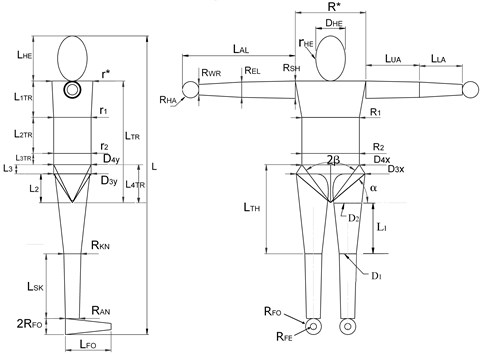When one represents via a mathematical model the HB one should successively solve specific problems: 1. Appropriate body decomposition – descriptions of the anthropometric points outlining segments and relevant characteristic lengths; 2. Generation of a proper 3D model that includes the decision which segment of the body shall be modelled with what geometrical body; 3. Analytical determination of the characteristics of the segments of interest; 5. Computer realization of the 3D model with the anthropometric data; 6. Verification of the computer-generated model by comparing the data obtained from the determination of the HB mass properties, using analytically derived results with those obtained based on computer realization; 7. Determination of the characteristics of interest of a particular part of the body, or the body as a whole, using the computer realization.

In the present article, we use the aforementioned recipe to study the mass-inertial properties of the HB via a combination of mathematical and computer modelling. For our study, we assume full-body symmetry to the midsagittal plane. Details of the exact dimensions, decomposition of the body for all segments except those of the thigh, which will be given in the following paragraph, can be found in .

The geometrical data needed for the determination of all lengths required is taken from a comprehensive representative anthropological investigation of the Bulgarian population  executed during the period 1989-1993. One has investigated 2435 males. The average values found in the above study are used to design a model, which characterizes the “average” Bulgarian man.

We model each segment of the HB through 3D geometrical forms as follows: (1) the torso is decomposed in three parts; (2) the upper part is modelled by a right reverted elliptical cone; (3) the middle and lower torso are shaped like an elliptical cylinder and an elliptical cylinder +reverted elliptical cone. The torso lower part is defined exactly as in : it extends from omphalion to iliospinale, with a plane passing through the iliospinales and concluding an angle of 37° with the sagittal plane. We consider the thigh, as divided into three elements. We did examine that question in detail in our previous article . Here, for the convenience of the readers, we just repeat a visualization of the way we do model the thigh in Fig. 2. The different segments of the thigh are shown in different colours. We represent the upper arm, lower arm and shank as a frustum of a cone and the hand is approximated as a sphere.

Fig. 2a) General idea for the 3D model of the human thigh; b) a computer realization of all the three geometrical parts embraced within the mathematical model of the thigh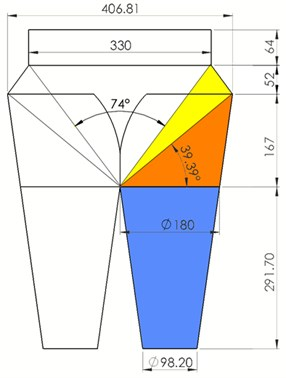a)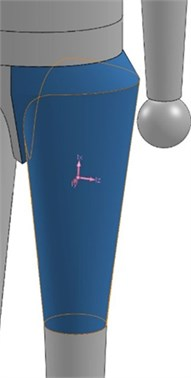b)

Knowing the anthropometric parameters of the segments, one can derive analytically all the properties of interest, such as volume, mass, and centre of mass and moments of inertia.

For instance, analytical expressions for two of the inertial moments for a frustum of an elliptic cone are given by the subsequent equations :

1
${I}_{XX}^{CM}=\frac{1}{240}\pi h\rho \left({R}_{2 }\left(4{h}^{2}+3{r}_{2}^{2}\right)\left(3{r}_{1}+2{R}_{1}\right)+3{r}_{2}\left(4{h}^{2}+{r}_{2}^{2}\right)\left(4{r}_{1}+{R}_{1}\right)-\frac{10{\left(h {r}_{2}\left(3{r}_{1}+{R}_{1}\right)+h {R}_{2}\left({r}_{1}+{R}_{1}\right)\right)}^{2}}{{r}_{1}\left(2{r}_{2}+{R}_{2}\right)+{R}_{1}\left({r}_{2}+2{R}_{2}\right)}+3{r}_{2}{R}_{2}^{2}\left(2{r}_{1}+3{R}_{1}\right)+3{R}_{2}^{3}\left({r}_{1}+4{R}_{1}\right)\right),$
2
${I}_{ZZ}^{CM}=\frac{1}{80}h\pi \rho \left(\left({r}_{1}^{3}\left(4{r}_{2}+{R}_{2}\right)+{r}_{1}^{2}{R}_{1}\left(3{r}_{2}+2{R}_{2}\right)$
$+{r}_{1}\left(4{r}_{2}^{3}+3{r}_{2}^{2}{R}_{2}+2{r}_{2}{R}_{2}^{2}+{R}_{2}^{3}+{R}_{1}^{2}\left(2{r}_{2}+3{R}_{2}\right)\right)\right)$
$+{R}_{1}\left({r}_{2}^{3}+2{r}_{2}^{2}{R}_{2}+3{r}_{2}{R}_{2}^{2}+4{R}_{2}^{3}+{R}_{1}^{2}\left({r}_{2}+4{R}_{2}\right)\right)\right).$

We perform a computer realization of the model in CAD software SolidWorks. Then we verify the computerized model by comparing the calculated results for the MIC of the segments of the body with the ones reported in . When the mass-inertial parameters of the segments are obtained, the corresponding characteristics of the whole body can be found assuming it to be in any position of interest.

As specified before, the fundamental postures of the body of significance for NASA are categorized in , as well as in -. Normally, eight principal body positions are of special interest. Here, due to space constraints, data will be shown for three of these positions: the so-called “standing position” (SP)– see Fig. 3(a), “standing, arms over head” (SAOH)– see Fig. 3(b), and “sitting, thighs elevated position” (STE) – see Fig. 3(c). In the remainder of the article, we comment on the computer-based simulation model realization and report data for characteristics of interest of the body in any of the stated postures.

## 3. Determination of mass-inertial characteristics in different body positions

The 3D CAD software SolidWorks is used to generate the model. This computer-generated model is validated by comparing its results with the analytical results we have obtained for segments of the body. The software procreates segment-by-segment data about volume, mass, centre of mass and moments of inertia, which provides confidence for using the model for calculating of these characteristics at different body positions. As stated above, we consider the SP, SAOH and STE positions. A laboratory coordinate system is defined, as shown in Fig. 3, for each of these positions. The axes coincide with the approximate body axes: the frontal ($x$), the sagittal ($y$), and the longitudinal ($z$) ones.

Fig. 3a) Standing position – subject stands erect with head oriented in the Frankfort plane and with arms hanging naturally at the sides as when measuring stature, b) standing, arms over head. Legs, torso, and head same as position 1; upper extremities raised over head, parallel to Y-axis; wrist axes parallel to X-axis; hands slightly clenched, c) sitting, thighs elevated position: thighs and forearms are placed parallel to the Z-axis, the upper arms, shanks, and spine are parallel to the Y-axis; the soles are parallel to the X-Z plane; wrist axes are parallel to Z-axis, and the head lies in Frankfort plane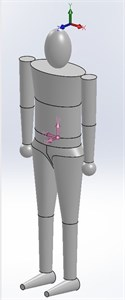a)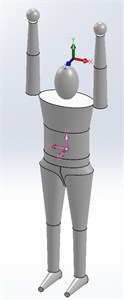b)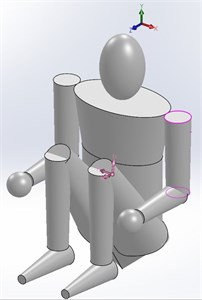c)

The mass inertial characteristics of the HB in these three positions are given in Tables 1, 2, and 3. Table 1 contains the data for the SP, Table 2 – for SAOH, and Table 3 – for the STE position.

A comparison of our new data with those from our previous study of the 16 segmental biomechanical model , , as well as with data from other literature sources are also reported in the Tables.

Naturally, all units as well as reference systems used in [1, 2, 4, 5, 8, 12, 13], has been transferred to the ones utilized in the current study.

The marks 50 % and 95 % which label the data of  and NASA  imply that this percent of measured data are beneath the value stated in the Table. We observe that our data are in good accord with those described in the literature.

Table 1Standing position

 Characteristic NASA Ref.  ChandlerRef.  SantschiRef.  HanavanRef.  Nikolova Ref.  Our data 50 % 95 % 50 % 95 % ${I}_{XX}$ [kg.cm2×103] 14.4 18.5 17.2 12.7 9.1 14.1 9.7 9.9 ${I}_{YY}$[kg.cm2×103] 129.2 163.4 118.9 116.0 116.2 161.9 105.3 108.0 ${I}_{ZZ}$ [kg.cm2×103] 144.5 182.3 134.0 129.5 122.3 171.1 112.0 117.0 Center of mass [cm] 80.2 84.7 72.3 78.7 80.0 83.8 74.6 71.8 Total mass [kg] 82.2 98.5 65.2 75.5 73.4 90.9 72.5 72.5 Height [cm] 179.9 190.1 172.1 176.3 175.5 185.7 171.5 171.5

 Characteristic NASARef.  SantschiRef.  HanavanRef.  NikolovaRef.  Our data 50 % 95 % 50 % 95 % ${I}_{XX}$[kg.cm2×103] 14.1 17.5 12.5 9.08 14.1 9.7 11.7 ${I}_{YY}$ [kg.cm2×103] 172.9 221.0 154.3 153.2 213.0 145.6 146.0 ${I}_{ZZ}$ [kg.cm2×103] 191.9 242.6 171.2 159.3 222.2 152.2 137.0 Center of mass [cm] 73.9 77.9 72.6 73.7 77.2 68.4 65.2

Table 3Sitting, thighs elevated position

 Characteristic NASARef.  ClauserRef.  NikolovaRef.  Our data 50 % 95 % ${I}_{XX}$[kg.cm2×103] 13.1 15.2 17.9 18.3 17.9 ${I}_{YY}$ [kg.cm2×103] 48.6 55.8 42.8 33.3 35.1 ${I}_{ZZ}$ [kg.cm2×103] 48.7 59.8 44.0 31.4 32.8 $L\left(x\right)$ [cm] 59.4 61.5 58.7 53.6 54.5 $L\left(y\right)$ [cm] 0 0 0 0 0 $L\left(z\right)$ [cm] 9.7 10.5 11.7 7.3 9.0

## 4. Conclusions

The current article presents the CAD design of a newly proposed 20-segmental biomechanical model of the HB of the average Bulgarian man. The model is an improvement over our previous model, which consisted of 16 segments. Obviously, the inertial moments depend, on the actual shape of the segment and the mass distribution in the body. In the existing models of the HB even the question of how the hip enters the torso and the appropriate division of the body in a part that belongs to the torso and another one belonging to the hip is not satisfactory achieved. The point is that one normally models the torso and the thigh with relatively simple geometrical figures that do not tailor continuously into each other, and which do even overlap. The current study presents some progress in this direction. The thigh consists of 3 parts – see Fig. 2. Modelling them with geometrical figures nearer to the actual form of these segments improves the model of the thigh and, thus, of the body.

In the current article we present data for the mass inertial parameters of the body of the average Bulgarian male in three essential positions – SP, SAOH, and STE – see Tables 1-3, where we also match our findings with other data reported in the literature. The specific anthropometric lengths needed to perform the calculations are taken from Ref. . The results obtained, and the procedure proposed in the present work allows us to claim that more realistic modelling of the shape of the HB is proposed that gives us confidence that this model could be used to calculate the mass inertial characteristics at any specific posture of the body.

Let us stress, that despite the model is applied to the average Bulgarian male, it is applicable to any gender, race, and for any specific person, given the suitable anthropometric measurements are made.

21 May 2021
Accepted
14 June 2021
Published
28 June 2021
SUBJECTS
Biomechanics and biomedical engineering
Keywords
3D human body modelling
biomechanics
mass-inertial characteristics
Acknowledgements

The financial support by the Bulgarian National Science Fund: Contract DN-07/5 “Study of anthropometric and mass-inertial characteristics of the Bulgarian men and women via mathematical models of the human body” is gratefully acknowledged.